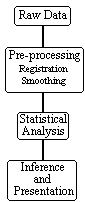### 6.1 Introduction

This chapter deals with the way in which the raw data from an fMRI experiment is analysed. The aim of such analysis is to determine those regions in the image in which the signal changes upon stimulus presentation. Although it is possible to devise many different techniques for detecting activation, if these techniques are to be used in practice it is necessary to know how much confidence can be placed in the results. That is to say, what is the probability that a purely random response could be falsely labeled as activation. This requires an understanding of the statistics behind the technique used.

Many of the statistically robust techniques used to analyse fMRI data have been developed from PET. These try to model the time course that is expected, and determine how well each pixel's temporal response fits this model. However, since fMRI experiments allow a much greater time resolution than PET, it is possible to carry out experiments which determine the order in which different cognitive events occur. Analysis of the data from such an experiment requires a 'non-directed' technique which makes few assumptions about the timings of the activation responses expected.

There are three stages to the analysis of the data from any fMRI experiment (Figure 6.1). Firstly there are the pre-processing steps, which can be applied to the data to improve the detection of activation events. These include registering the images, to correct for subject movement during the experiment, and smoothing the data to improve the signal to noise ratio. Next, the statistical analysis, which detects the pixels in the image which show a response to the stimulus, is carried out. Finally the activation images must be displayed, and probability values, which give the statistical confidence that can be placed in the result, quoted.##### Figure 6.1 Steps involved in the processing of fMRI data

In order to analyse the data from the experiments explained in Chapter 7, a suite of programs were written, which implement existing and novel analysis techniques for carrying out the steps outlined above. These techniques are described in detail in the following sections.

Previous Section | Contents | Next Section